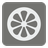Even the Last
ElementaryYou are given an array of integers. You should find the sum of the integers with even indexes (0th, 2nd, 4th...). Then multiply this summed number and the final element of the array together....

For an empty array, the result will always be 0 (zero).

A list of integers.

The number as an integer.

```checkio([0, 1, 2, 3, 4, 5]) == 30
checkio([1, 3, 5]) == 30
checkio() == 36
checkio([]) == 0
```

Indexes and slices are important elements of coding. This will come in handy down the road!

0 ≤ len(array) ≤ 20
all(isinstance(x, int) for x in array)
all(-100 < x < 100 for x in array)

You should be an authorized user in order to see the full description and start solving this mission.